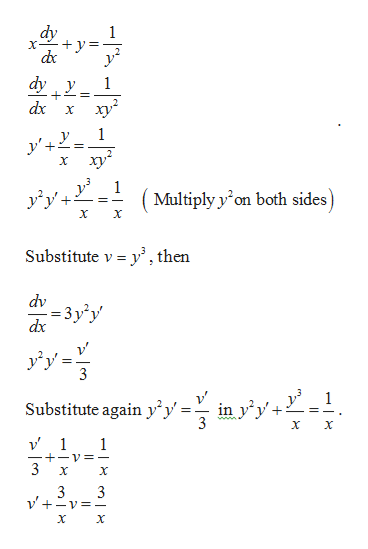# Solve the given differential equation by using an appropriate substitution. The differential equation is a Bernoulli equation.x(dy/dx) + y = 1/y2

Question
540 views

Solve the given differential equation by using an appropriate substitution. The differential equation is a Bernoulli equation.

x(dy/dx) + y = 1/y2

check_circle

Step 1

Consider the given differential equa...help_outlineImage Transcriptionclosedy X- dy y 1 dx y' 1 X y1 Multiply y on both sides) X Substitute v y, then dv 3y y dx y'y' 3 1 in y'y Substitute again y^y 3 1 1 +-v=- 3 3 fullscreen

### Want to see the full answer?

See Solution

#### Want to see this answer and more?

Solutions are written by subject experts who are available 24/7. Questions are typically answered within 1 hour.*

See Solution
*Response times may vary by subject and question.
Tagged in

### Math